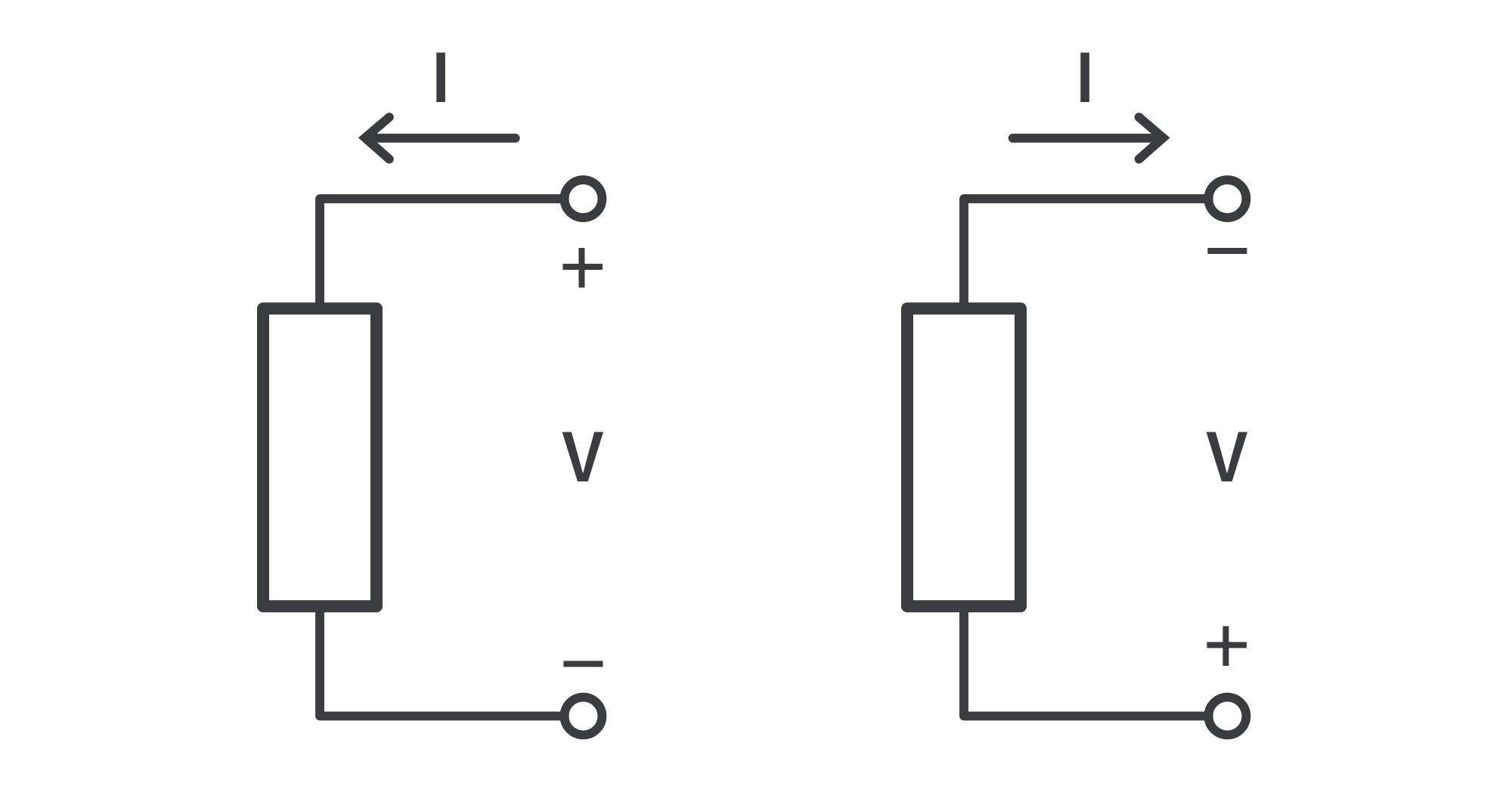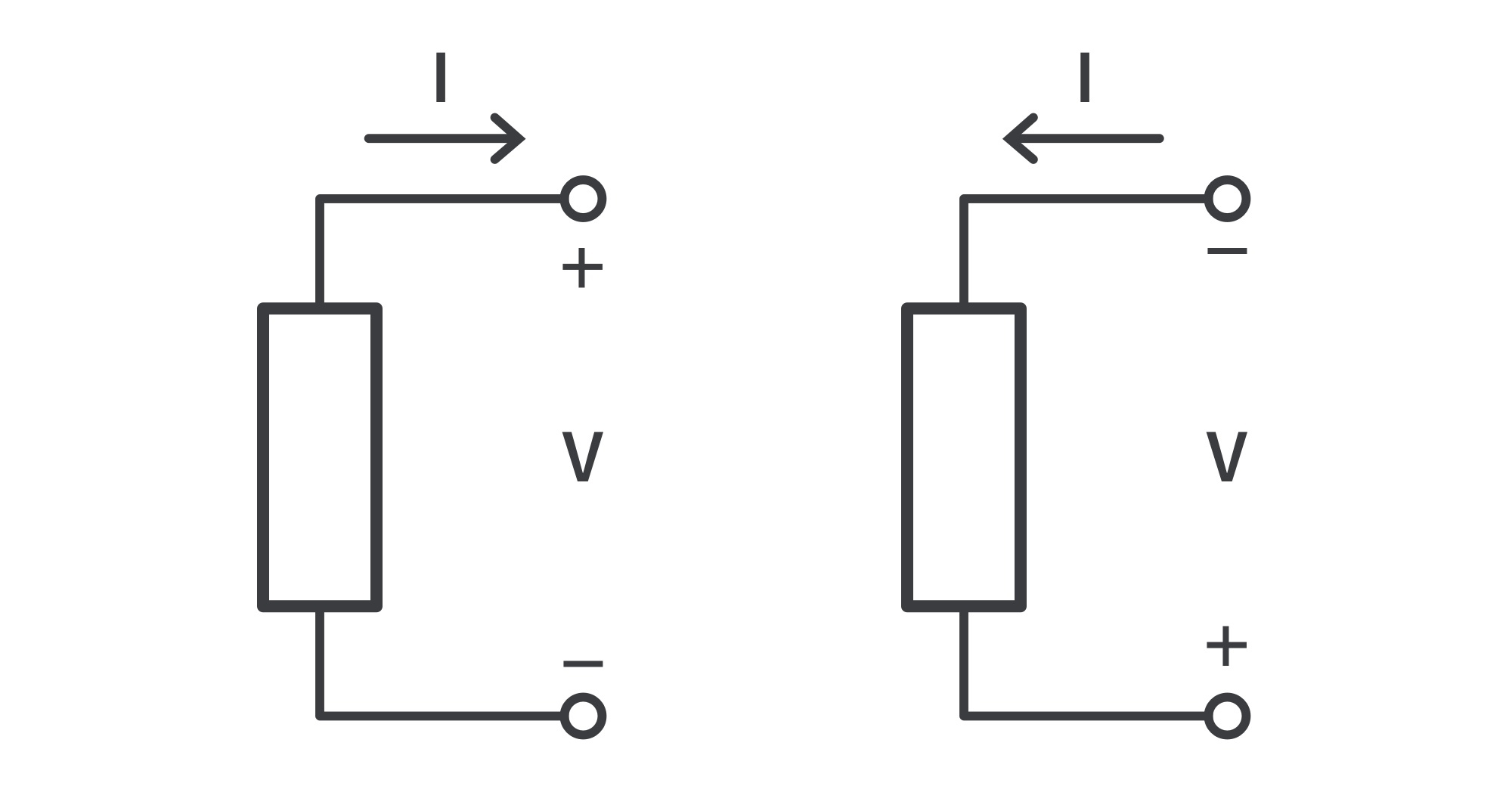Study Guide

# DC Circuits | Basic Concepts

Charge and Current

## Charge

• Charge is an electrical property of the atomic particles of which matter consists, measured in coulombs (C).
• Notes on electric charge:
1. The coulomb is a large unit for charges. In 1 C of charge, there are 1/(1.602 x 10-19) = 6.24 x 1018 electrons; realistic or laboratory values of charges are on the order of pC, nC, or μC.
2. The only charges observed to occur in nature are integral multiples of the electronic charge, e = -1.602 x 10-19 C.
3. The law of conservation of charge states that charge can neither be created nor destroyed, only transferred. Thus, the algebraic sum of the electric charges in a system does not change.
• When a conducting wire of several atoms is connected to a source, the charges are compelled to move; positive charges move in one direction while negative charges move in the opposite direction. This motion of charges creates an electric current.
Charge and Current

## Current

• Electric current is the time rate of change of charge, measured in amperes (A).

• The relationship between current i, charge q, and time t is

where current is measured in amperes (A), and 1 ampere = 1 coulomb/second

• The charge transferred between time to and t is
• A direct current (dc) flows only in one direction and can be constant or time-varying.

• An alternating current (AC) is a current that changes direction with respect to time.

• Andre-Marie Ampere (1775–1836), a French mathematician and physicist, laid the foundation of electrodynamics. He defined the electric current and developed a way to measure it in the 1820s. The unit of electric current, the ampere, was named after him.

## Voltage

• Voltage (or potential difference) is the energy required to move a unit charge from a reference point (−) to another point (+), measured in volts (V).
• The voltage vab between two points a and b in an electric circuit is the energy (or work) needed to move a unit charge from b to a; mathematically

where w is energy in joules (J) and q is charge in coulombs (C). Hence, 1 Volt = 1 Joule/Coulomb = 1 Newton-meter/Coulomb

• Voltage is measured in volts (V) in honor of Alessandro Antonio Volta (1745–1827), an Italian physicist who invented the first voltaic battery.

• vAB can be interpreted in two ways: (1) Point a is at a potential vBA above point b; (2) point b is -vAB above point a:

• It follows logically that in general:

• In other words, a voltage drop from a to b is equivalent to a voltage rise from b to a.
Power and Energy

## Power

• Power is the time rate of expending or absorbing energy, measured in watts (W); mathematically:

where p is power in watts (W), w is energy in joules (J), and t is time in seconds. This power is time-varying, called the instantaneous power.

• If the power has a + sign, power is being delivered to or absorbed by the element; if it has a − sign, power is being supplied by the element.

• Passive sign convention: when the current enters through the positive terminal of an element and p = +vi. If the current enters through the negative terminal, p = -vi.Two cases of an element with a supplying power of iv (p=-|vi|)

• The algebraic sum of power in a circuit, at any instant of time, must be zero and so the total power supplied to the circuit must balance the total power absorbed. An absorbing power of −W is equivalent to a supplying power of +W. In general,
Power and Energy

## Energy

• Energy is the capacity to do work, measured in joules (J).

• The energy absorbed or supplied by an element from time to to time t is

• Electric power utility companies measure energy in Watt-hours (Wh), where 1 Wh = 3,600 J
Circuit Elements

## Sources

• Voltage or current sources deliver power to the circuit connected to them. There are two kinds of sources: independent and dependent.
• An ideal independent source is an active element that provides a specified voltage or current that is completely independent of other circuit elements.
• Independent Voltage sources deliver to the circuit whatever current is necessary to maintain terminal voltage.
• Batteries are made up of voltaic cells which provide a steady DC voltage at the output terminals. Voltage output and current rating are determined by the electrodes and rechargeability by the type of cells.

• Independent Current sources provide a specified current completely independent of the voltage across the source. The current source delivers to the circuit whatever voltage is necessary to maintain the designated current.

• An ideal dependent/controlled source is an active element in which the source quantity is controlled by another voltage or current.

• Since the control of the dependent source is achieved by a voltage or current of another circuit element, and the source can be voltage or current, there are four possible types of dependent sources:

1. A voltage-controlled voltage source (VCVS).
2. A current-controlled voltage source (CCVS).
3. A voltage-controlled current source (VCCS).
4. A current-controlled current source (CCCS).
• An ideal voltage source (dependent or independent) will produce any current required to ensure that the terminal voltage is as stated.

• An ideal current source will produce the necessary voltage to ensure the stated current flow.

• An ideal source could in theory supply an infinite amount of energy.

Circuit Elements

## Resistors

• The main function of resistors in any circuit is to limit the amount of current or to produce a desired drop in voltage.

• The resistance R of an element denotes its ability to resist the flow of electric current; it is measured in ohms (Ω).

• Resistors are manufactured in a wide range of ohmic values. While the power rating is determined mainly by its physical size, there is no direct correlation between the size of a resistor and its resistance value.

• The equivalent resistance of any number of resistors connected in series is the sum of the individual resistances.
• The equivalent resistance of two parallel resistors is equal to the product of their resistances divided by their sum. For the general case of N resistors connected in parallel,
• Ohm’s law: the voltage v across a resistor is directly proportional to the current i flowing through the resistor ⟹ v=iR

• Power dissipated by a resistor: p=iv=v2/R

Circuit Elements

## Capacitors

• A capacitor is a passive element designed to store energy in its electric field; it consists of two conducting plates separated by an insulator (or dielectric). The plates may be aluminum foil while the dielectric may be air, ceramic, paper, or mica.

• Capacitance (C) is the ratio of the charge on one plate of a capacitor to the voltage difference between the two plates: C=q/V, measured in farads (F) in honor of Michal Faraday (1791–1867), an English chemist and physicist.

• Capacitance actually depends on the physical dimensions of the capacitor:

where A is the surface area of each plate, d is the distance between the plates, and ϵ is the permittivity of the dielectric material between the plates.

• Important properties of a capacitor:
1. A capacitor is an open circuit to dc. However, if a battery is connected across a capacitor, the capacitor charges.

2. The voltage on a capacitor cannot change abruptly.

3. The ideal capacitor does not dissipate energy.

4. A real, non-ideal capacitor has a parallel-model leakage resistance.

• Capacitors are used extensively in electronics, communications, computers, and power systems; in the tuning circuits of radio receivers, and as dynamic memory elements in computer systems.
• The total capacitance for capacitors connected in series is
• The total capacitance for capacitors connected in parallel is
• Current-voltage relation:
• Voltage-current relation:
• Energy stored in the electric field that exists between the plates of the capacitor:
Circuit Elements

## Inductors

• An inductor is a passive element designed to store energy in its magnetic field. Any conductor of electric current has inductive properties and may be regarded as an inductor.
• To enhance the inductive effect, a practical inductor is usually formed into a cylindrical coil with many turns of conducting wire.
• Inductance L is the opposition to the change of current flowing through an inductor, measured in henrys (H) in honor of Joseph Henry (1797–1878), an American physicist who discovered inductance and constructed an electric motor.
• Inductance of an inductor depends on its physical dimension and construction. For a solenoid,

where N is the number of turns, ℓ is the length, A is the cross-sectional area, and u is the permeability of the core.

• Inductors may be fixed or variable. The core may be made of iron, steel, plastic, or air. The terms coil and choke are also used for inductors.
• Important properties of an inductor:
1. An inductor acts like a short circuit to dc.

2. Current through an inductor cannot change instantaneously.

3. The ideal inductor does not dissipate energy.

4. A practical, non-ideal inductor has a significant resistive component.

• Inductors find numerous applications in electronic and power systems. They are used in power supplies, transformers, radios, TVs, radars, and electric motors.
• The total inductance for inductors connected in series is